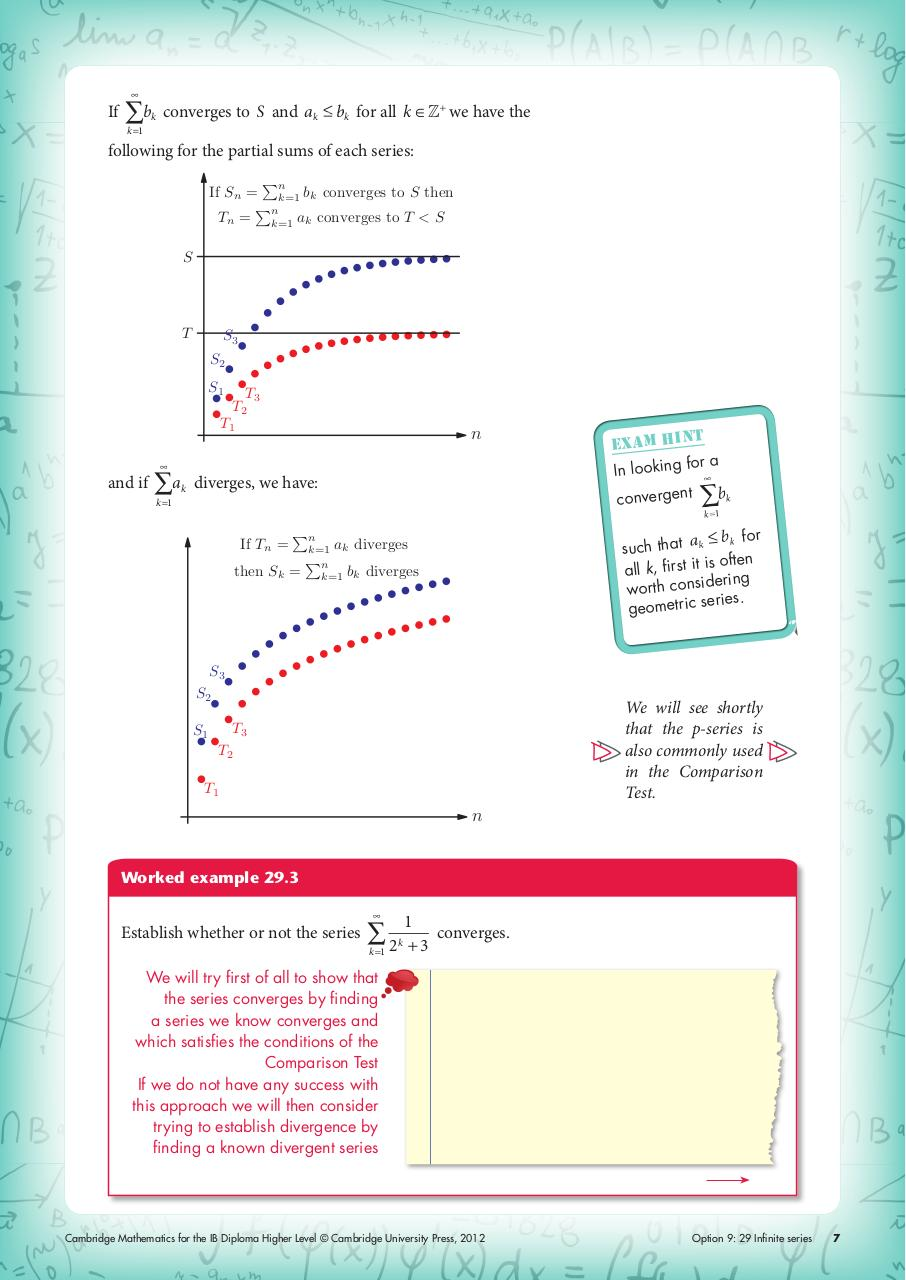# ch12.pdfPage 1 2 3 45626

#### Text preview

If

∑b

k

converges to S and ak

bk for all k ∈Z + we have the

k =1

following for the partial sums of each series:
n
If Sn = k=1 bk converges to S then
n
Tn = k=1 ak converges to T &lt; S
S

T

S3
S2
S1

T3
T2

T1

n

and if

∑a

k

diverges, we have:

convergent

k =1

If Tn =

n

then Sk =

∑b

k

k =1

a bk for
such that k
is often
all k, first it
dering
worth consi
ries.
geometric se

k=1 ak diverges
n
k=1 bk diverges

S3
S2
S1

t
exam hin
ra
In looking fo

We will see shortly
that the p-series is
also commonly used
in the Comparison
Test.

T3
T2

T1

n
Worked example 29.3

Establish whether or not the series

∑2
k =1

k

1
converges.
+3

We will try first of all to show that
the series converges by finding
a series we know converges and
which satisfies the conditions of the
Comparison Test
If we do not have any success with
this approach we will then consider
trying to establish divergence by
finding a known divergent series

Cambridge Mathematics for the IB Diploma Higher Level © Cambridge University Press, 2012

Option 9: 29 Infinite series

7